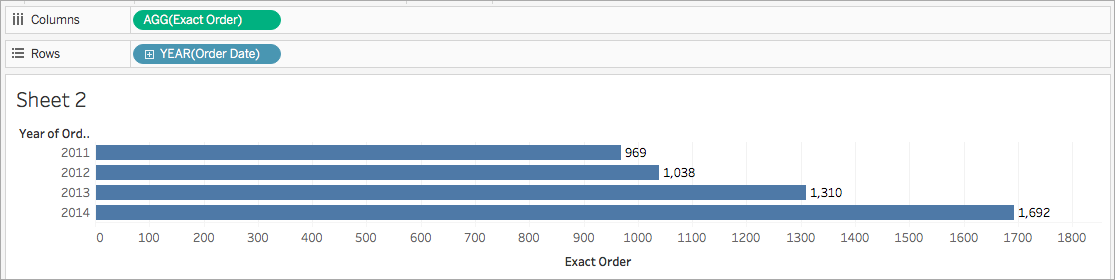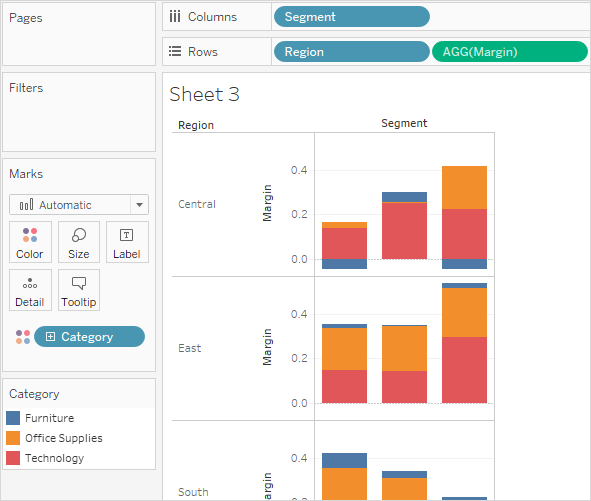# Tableau 中的聚合函数

## 为何使用聚合函数

`COUNTD(Order ID)`## Tableau 中的可用聚合函数

#### 定义

ATTR

`ATTR(expression)`

AVG

`AVG(expression)`

COLLECT

`COLLECT (spatial)`

`COLLECT ([Geometry])`

CORR

`CORR(expression 1, expression2)`

CORR 可用于以下数据源：

• Tableau 数据提取（您可以从任何数据源中创建数据提取）
• Cloudera Hive
• EXASolution
• Firebird（版本 3.0 及更高版本）
• IBM PDA (Netezza)
• Oracle
• PostgreSQL
• Presto
• SybaseIQ
• Vertica

`{CORR(Sales, Profit)}`

COUNT

`COUNT(expression)`

COUNTD

`COUNTD(expression)`

COVAR

`COVAR(expression 1, expression2)`

COVAR 可用于以下数据源：

• Tableau 数据提取（您可以从任何数据源中创建数据提取）
• Cloudera Hive
• EXASolution
• Firebird（版本 3.0 及更高版本）
• IBM PDA (Netezza)
• Oracle
• PostgreSQL
• Presto
• SybaseIQ
• Vertica

`COVAR([Sales], [Profit])`

COVARP

`COVARP(expression 1, expression2)`

COVARP 可用于以下数据源：

• Tableau 数据提取（您可以从任何数据源中创建数据提取）
• Cloudera Hive
• EXASolution
• Firebird（版本 3.0 及更高版本）
• IBM PDA (Netezza)
• Oracle
• PostgreSQL
• Presto
• SybaseIQ
• Vertica

`COVARP([Sales], [Profit])`

MAX

`MAX(expression)`

MEDIAN

`MEDIAN(expression)`

• 访问
• Amazon Redshift
• HP Vertica
• IBM DB2
• IBM PDA (Netezza)
• Microsoft SQL Server
• MySQL
• SAP HANA

MIN

`MIN(expression)`

PERCENTILE

`PERCENTILE(expression, number)`

• 非旧版 Microsoft Excel 和文本文件连接。

• 数据提取和纯数据提取数据源类型（例如 Google Analytics（分析）、OData 或 Salesforce）。

• Sybase IQ 15.1 及更高版本的数据源。

• Oracle 10 及更高版本的数据源。

• Cloudera Hive 和 Hortonworks Hadoop Hive 数据源。

• EXASolution 4.2 及更高版本的数据源。

STDEV

`STDEV(expression)`

STDEVP

`STDEVP(expression)`

SUM

`SUM(expression)`

VAR

`VAR(expression)`

VARP

`VARP(expression)`

## 创建聚合计算

1. 在 Tableau Desktop 中，连接到 Tableau 附带的示例 - 超市已保存数据源。

2. 导航到工作表，并选择“分析”>“创建计算字段”

3. 在打开的计算编辑器中，执行以下操作：

• 将计算字段命名为“Margin”（利润）

• 输入以下公式：

```IIF(SUM([Sales]) !=0, SUM([Profit])/SUM([Sales]), 0)```

注意：您可以使用函数引用来查找聚合函数和其他函数（如此示例中的逻辑 IIF 函数），并将其添加到计算公式。有关详细信息，请参见在计算编辑器中使用函数引用

• 完成后，单击“确定”

新的聚合计算将出现在“数据”窗格中的“度量”下。就像其他字段一样，您可以在一个或多个可视化项中使用该字段。

注意：聚合计算始终为度量。

当将“Margin”（利润）放在工作表中的功能区或卡上时，它的名称将更改为“AGG(Margin)”，表示它是聚合计算，并且无法进一步聚合。## 聚合计算的规则

• 任何聚合计算中不得同时包括聚合值和解聚值。例如，SUM(Price)*[Items] 不是有效的表达式，因为 SUM(Price) 已聚合，而 Items 则没有。不过，SUM(Price*Items) 和 SUM(Price)*SUM(Items) 均有效。

• 表达式中的常量可根据情况充当聚合值或解聚值。例如：SUM(Price*7) 和 SUM(Price)*7 均为有效的表达式。

• 所有函数都可用聚合值进行计算。但是，任何给定函数的参数必须或者全部聚合，或者全部解聚。例如：MAX(SUM(Sales),Profit) 不是有效的表达式，因为 Sales 已聚合，而 Profit 则没有。不过，MAX(SUM(Sales),SUM(Profit)) 为有效的表达式。

• 聚合计算的结果始终为度量。

• 与预定义聚合一样，聚合计算可正确地进行总计计算。有关详细信息，请参见“总计”。

## 另请参见

Tableau 中的数据聚合(链接在新窗口中打开)

Tableau 中的函数

Tableau 函数（按类别）(链接在新窗口中打开)

Tableau 函数（按字母顺序）